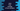# Java program to find the average of two numbers## Java program to find the average of two numbers:

In this post, we will learn how to find the average value of two different numbers in Java. We will take the values as inputs from the user and print out the average.

We will also learn how to find the average of two numbers using a different method.

With this program, you will learn how to take user inputs in Java, how to do basic mathematical calculations and how to print values on console.

### Algorithm:

It is easy to find the average of two numbers. If you know how to do that, you can write a program easily. Our program will follow the following steps:

• Take the numbers as inputs from the user.
• Find the sum of the numbers.
• Divide the sum by 2 to find the average value.
• Print the average value.

## Java program to print the average value of two numbers:

Below is the complete program:

``````import java.util.Scanner;

public class Main {

public static void main(String[] args) {
int first, second;
float average;

Scanner sc = new Scanner(System.in);

System.out.println("Enter the first number: ");
first = sc.nextInt();

System.out.println("Enter the second number: ");
second = sc.nextInt();

average = (float) (first + second) / 2;

System.out.println("Average value: " + average);
}
}``````

Here,

• first and second are two integer variables to hold the first and second numbers.
• average is to store the average value. This is a floating point variable.
• sc is a Scanner object. We need an object of Scanner class to read user input values.
• It asks the user to enter the first and the second number, read them and stores in the variables.
• Once both numbers are entered, it finds the average by dividing the sum by 2. We are converting it to a float value.
• The last line is printing the average value.

If will print output as like below:

``````Enter the first number:
120
Enter the second number:
125
Average value: 122.5``````

## Find the average for float inputs:

The above program will work only for integer numbers. If you want to take float values as input, you need to change this to something like below:

``````import java.util.Scanner;

public class Main {

public static void main(String[] args) {
float first, second, average;
Scanner sc = new Scanner(System.in);

System.out.println("Enter the first number: ");
first = sc.nextFloat();

System.out.println("Enter the second number: ");
second = sc.nextFloat();

average = (first + second) / 2;

System.out.println("Average value: " + average);
}
}``````

It is almost similar to the above program. The only difference is that we are using float variables for the user input numbers. To read the values, we need to use nextFloat method.

If you run this program, it will give output as like below:

``````Enter the first number:
120.44
Enter the second number:
133.45
Average value: 126.945``````

## Find the average using a different function:

We can use a separate function to find the average value. This function will take the numbers as inputs and it will return the average. The advantage of using a separate function is that we can call it from different places in an application. For example, you can call this function from class A and class B. If you want to make any change to it, you can do it at one place.

``````import java.util.Scanner;

public class Main {

public static float findAverage(float x, float y) {
return (x + y) / 2;
}

public static void main(String[] args) {
float first, second;
Scanner sc = new Scanner(System.in);

System.out.println("Enter the first number: ");
first = sc.nextFloat();

System.out.println("Enter the second number: ");
second = sc.nextFloat();

System.out.println("Average value: " + findAverage(first, second));
}
}``````

Here, we created a different function findAverage that takes two float values as inputs and returns the average value.